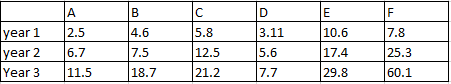# CAT 1996 Question Paper

Instructions

Direction for questions: Answer the questions based on the following information. The data given in the table shows the investment details in country ‘Fortune Land’ of companies A, B, C, D, E and F. Figures in the table are in US dollars in billions.Question 1

Question 2

Question 3

Question 4

Question 5

# By what percentage is the investments of companies D,E,F greater than the investments of companies A, B, C in year 2?

Instructions

For the following questions answer them individually

Question 6

Question 7

# Which of the following values of x do not satisfy the inequality $$(x^2 - 3x + 2 > 0)$$ at all?

Instructions

Direction for questions 108 and 109: Answer the questions based on the following information. In a locality, there are five small cities: A, B, C, D and E. The distances of these cities from each other are as follows. AB = 2 km AC = 2km AD > 2 km AE > 3 km BC = 2 km BD = 4 km BE = 3 km CD = 2 km CE = 3 km DE > 3 km

Question 8

Question 9

# If a ration shop is to be set up within 3 km of each city, how many ration shops will be required?

Instructions

For the following questions answer them individually

Question 10

OR